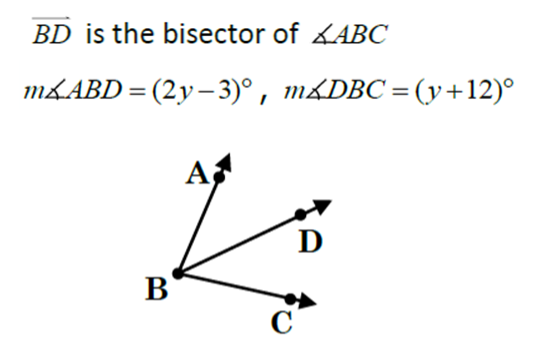GeoB blast 1
Find x only *
1 point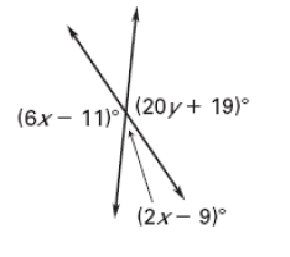Complementary angles *
2 points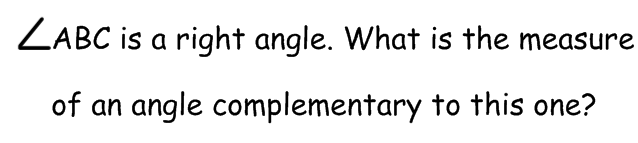B is the midpoint of AC. Find x *
1 point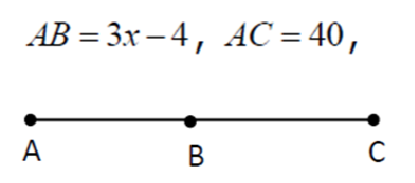Find angle f only *
2 pointssegment addition (little + little = big) Find x. *
2 points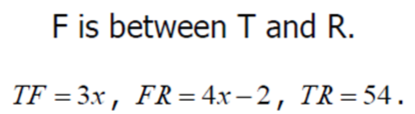two angles are supplementary. One angle is 90 degrees. What's the measure of the second? *
2 points
Find x. *
1 point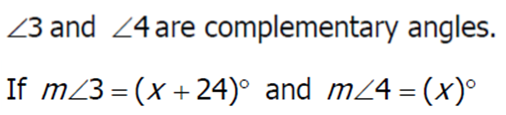Find x. Angle addition postulate (L + L = B) *
1 point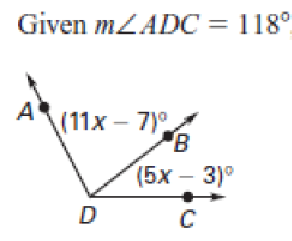Choose which bests describes these angles *
2 points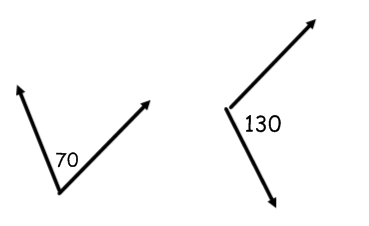Find angle y *
2 pointsThese two angles are... *
2 points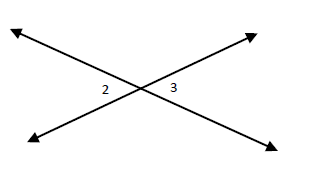Find x *
1 point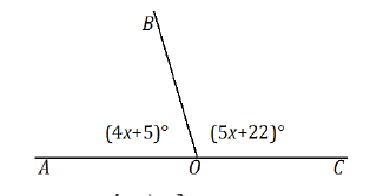Find the measure of angle T. Angle T and U have the same measure. *
2 points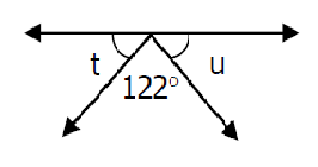segment addition (little + little = Big) *
2 points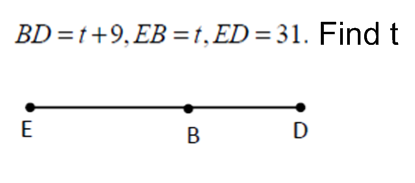Segment Addition (Little + Little = BIG).. Find x *
2 pointsLast Name and Period *